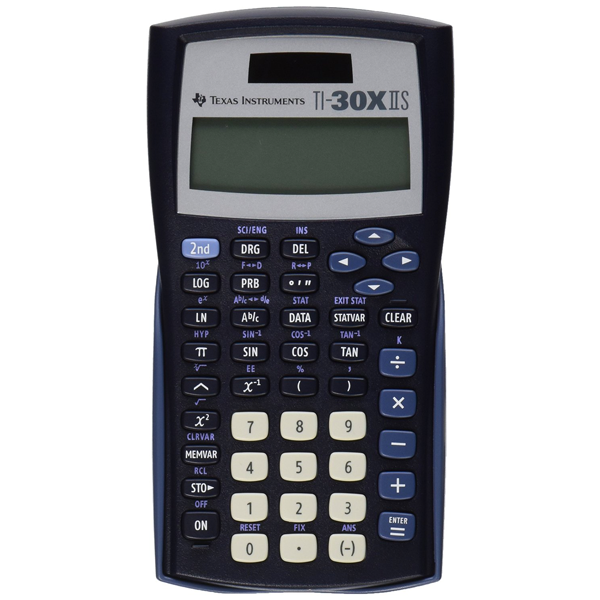# TI 30XIIS Scientific CalculatorSKU:
1148152

# TI 30XIIS Scientific Calculator

 \$12.98
Description:

### Key features:

• Two-line display
• Fraction features
• Conversions
• Basic scientific and trigonometric functions
• Edit, cut, and paste entries
• Solar and battery powered
• One- and two-variable statistics
• Exam acceptance: The TI-30XIIS scientific calculator is approved for use on SAT, ACT, and AP exams.

### Ideal for:

• General math
• Pre-Algebra
• Algebra 1 and 2
• Geometry
• Statistics
• General science

• Broaden exploration of math and science concepts: Review previous entries and look for patterns. A two-line display shows entries on the top line and results on the bottom line.
• Perform advanced scientific functions: Features one- and two-variable statistics, recognizes π as a symbol in radian mode, and provided menus that meet your calculating needs.
• Perform operations with fractions and mixed numbers: Adds, subtracts, multiplies, and divides fractions entered in traditional numerator/denominator format.
• SKU:
1148152
Description:

### Key features:

• Two-line display
• Fraction features
• Conversions
• Basic scientific and trigonometric functions
• Edit, cut, and paste entries
• Solar and battery powered
• One- and two-variable statistics
• Exam acceptance: The TI-30XIIS scientific calculator is approved for use on SAT, ACT, and AP exams.

### Ideal for:

• General math
• Pre-Algebra
• Algebra 1 and 2
• Geometry
• Statistics
• General science